# Lie Groups Simplified: the Symmetry Underlying the Laws of Physics

The laws of physics, to the amateur science enthusiast, can sometimes seem like a disparate bunch of equations without any real underlying connection. That doesn’t seem good enough, so you dig a little deeper, and soon discover that there is this thing called Lie Theory, with Lie Groups and Lie Algebras. You want to find out what all this is, and the only things you can find just give you another bunch of equations and leave it there as if those unexplained symbols are good enough for you to understand. Well, I’m at that point, and here I am going to talk about Lie Theory and its connection to physics in hopes that explaining things simply and intuitively will help me to better understand it – and if that helps any readers understand it better, that’s good, too.

### Basics of Group Theory

Let’s start by analyzing what a group is. A group is just a bunch of things (called elements) together in a larger thing (called a set) where, combining to elements of that set gives you another element of that set. For instance, we can say that the integer numbers (-, …, -5, -4, -3, -2, -1, 0, 1, 2, 3, 4, 5, …, ) are a set. Each number is an element of the bigger thing we call the ‘set of all integers.’ We can combine the elements using addition, and with that we will always get another integer number:

0 +1 = 1
0 + 100 = 100
3 + 5 = 8
177 + 20 = 197

And so on. You could sit here all day, adding two integer numbers, and you will always get another integer number. This is called closure (under that particular operation, addition in the case of integer numbers), and a set (like the integer numbers) where this is a property is called a closed set – it’s closed because you can never escape it by performing your operation and you cannot add anything new to the set by performing your operation on an element of the set with some outside thing (i.e. if you add 10 to the outside thing 3.1415926 you do not get an integer number).

Another interesting fact you an see from the first two examples above is that adding 0 to anything always gives you the same thing back. You an add 0 to any integer number you can think of and it will always give you that same integer number back. This is why 0 is, in the integer group, called the identity element – it is the element that, when you perform the group operation (addition) using any other element of the group with it, you will always get that same element back. This is another feature of groups: they always have an identity element. The identity element can also always be obtained by performing the group operation (addition, in this case) on an element an its inverse. In the case of the integers, the inverse is the negative version of the number:

1 + (-1) = 0
89 + (-89) = 0

Once again, you can sit here all day adding elements to their inverse and you will always get zero (the identity element). A group also has the property that it doesn’t matter how many times you perform the group operation (addition with integers) you will always get another element of the group:

6 + 4 = 10
831 + 86 = 917
917 + 10 = 927

You may also notice from this that we can push together these two groupwise things:

(6 + 4) + (831 + 86) = 10 + 917 = 927
6 + (4 + 831) + 86 = 6 + 835 + 86 = 927

And here, this also tells us that it doesn’t matter how we squish the numbers together, we still get the same thing. This is called associativity. This might seem trivial, but it is important.

All of this can apply to more than just mathematics, as well. Take, for instance, if you have a picture that’s been cut into 3 pieces. We can call these 3 pieces of the picture the elements and all of the pieces of the picture the set (i.e. a set of picture pieces). If we tape together two pieces of the pictures (the group operation) then we end up with another piece of the picture, so it’s closed (taping all three pieces together obviously gives you back the full picture, but it’s valid to call that an element of the set). If we tape the top third to the middle before we tape the bottom third to the middle we’ll get the same thing as if we tape the bottom third to the middle first, so it’s associative. If we take the bottom third and tape it to nothing, we still have just the bottom third, so there is an identity element. We could say that the inverse is removing a piece of the picture (this isn’t rigorously correct, but this is just an illustration) and so we end up with the identity element of “not taping two pieces together” (taping and then untaping is the same as not taping at all).

One thing you might notice here, with the picture example, that’s different from the integers is that it matters in what order we tape the pictures together. If I tape the top third onto the bottom of the middle and the bottom third onto the top of the middle, I get something different than if I tape the top onto the top and the bottom onto the bottom. This is different from the integer numbers, where if I do:

2 + 3 = 5 = 3 + 2

It doesn’t matter if the 2 or the 3 comes first, you still end up with 5. This is the commutative property. The “Picture” Group (the set of picture thirds under the “tape-together” operation) is non-commutative. A Group that is commutative, like the integer numbers, is what is known as an Abelian Group, named after Norwegian mathematician Niels Henrik Abel. If the Group is an Abelian Group, then the operation (like addition) is said to have symmetry.

A group is defined by the four axioms I discussed:

A Group is closed
A Group has associativity
A Group has an identity element (like 0 for the integer numbers)
A Group is invertible (like a positive integer number having a negative integer number)

But, just like communativity, Groups can have other properties. This is where things can get more conceptually and mathematically complicated. What we talked about so far is pretty simple – almost trivial – but the other properties that Groups need to have before they can be considered Lie Groups are more difficult to define without some background knowledge about vectors, linear transformations, and metrics. And so, I will give an extremely brief overview of what is relevant for our purposes.

### Overview of Linear Algebra

A vector is very abstract, but I will talk about particular kinds of vectors that are easier to understand and that you have likely encountered before. A vector, then, is something that has a magnitude and direction. When you are driving, you would express this is a vector: you are moving at 60 kilometers per hour (the magnitude) heading northeast (the direction). You could represent this as an arrow with its length representing your speed (60 km/h) and its orientation representing the direction you’re moving (northeast).If we want to know about the speed of something over a given space (rather than just your car), then we can think about multiple vectors located over some space. Consider a flowing river: it is not a single object moving from one place to another. The “river” remains where it is, but the that makes up the river is moving. And it is moving at some speed everywhere. And so, if we are standing next to the river, we can say that the river has some speed in the direction of flow 3 feet out into the river, 4 feet out into the river, 5 feet out into the river, etc. as well as at any place in between. The speed at the places in front of you can also be represented as vectors, with the arrow length representing the speed of the river’s flow and the orientation representing the direction of the river’s flow.Going back t our car example, though: if we want to calculate the length and orientation of this arrow representing your speed and direction, we need to come up with some way of easily representing it. The way we do this is by saying, if the arrow is pointing northeast, how much of that northeast direction is just east and how much is just north? In other words, I want to represent your velocity (velocity is what we call the vector representing speed and direction) as how fast you are moving in just the eastward direction and how fast you are moving in the northward direction and then add the two together to get your northeast direction. The pure eastwardnesss of your velocity and the pure northwardness of our velocity are called the components of your velocity – the east component and the north component. More abstractly, if we use a Cartesian coordinate system, we want to know the x-component (Vx in the above image) and the y-component (Vy in the above image) of your velocity. Now, if you are in an airplane, we might also want to add the up-down component of your velocity: the z-component. This means we can have vectors in 3-dimensions as well. It can also go to 4, 5, 6, 7 and so on up to infinity dimensions. What’s important in physics, though, are vectors in 4 dimensions (3 dimensions for space and 1 for time).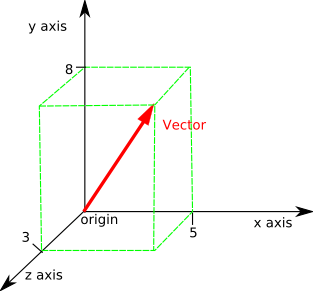Now, if we want to calculate a change in your velocity, we need to do what is called a vector transformation. To do this, you want to multiply your x-component by some number that represents the change in your velocity in the eastward direction, and we want to multiply your y-component by some number that represents the change in your velocity in the northward direction (the same could go for the z-component and so on up to an arbitrary number of dimensions, but I’ll stick with lower dimensions for illustrative purposes). That’s easy enough, you just multiply both components by however much each component changes. If you turn more eastward, you will gain eastward motion, so you multiply the x-component by some number bigger than 1; at the same time, you lose northward motion, so you multiply the y-component by some number less than 1. As you can see here, multiplying the component by a higher number gives you more of that component: this means that they are covariant – the number you use to multiply and the component of your vector vary in the same direction (as one gets bigger, so does the other; as one gets smaller, so does the other).

But what about if I am driving through an intersection that goes across the northeastward road you are diving down, but I am driving due east at 50 km/h? How would I calculate your velocity from my point of view? Obviously, for me, your eastward velocity will appear smaller to me, since I am also driving east. In fact, since my 50 km/h speed in the eastward direction is greater than the eastward component of your northeast speed, to me, it will look like you’re going west (i.e. I will be moving eastward faster than you are). How can it be that you are traveling both northeast (from your point of view) and north-northwest (from my point of view)?

Well, let’s think about what’s meant by “point of view.” So far, I’ve been priveliging the cardinal directions (North, East, South, West), which are helpful for thinking about the distance between two places, but less helpful when thinking about relative velocities. To do this, I would want to privilege my own point of view. Instead of my y-component pointing north, I’d instead say my y-component is pointing straight ahead and my x-component is pointing at a right angle from straight ahead, pointed to my right. Now my velocity vector just is the y-component (the x-component is zero). Now I can say what your velocity is relative to me; conversely, it’s just as valid for you to say what my velocity is relative to you. If we want to switch between these two points of view, then we’ll have to rotate the axes from one point of view to the other. This is called a change of basis.Left: transformation that shifts the direction of the vector P to a new direction in P` Right: transformation that rotates the basis vectors by some angle θ

This can be done for the river example from earlier, which would represent a vector field: a set or coordinates where every point in the coordinate plane can be represented by a vector (e.g. the velocity of the river’s flow at every point in the river). If I go to the other side of the river, then relative to me, the river’s flow is reversed. This can also be represented by a linear transformation.

Back to the traveling car example (but this could be applied to the vector field as well): the way to do a linear transformation is by first assigning some way of measuring each component (the x-component and y-component). We already are using km/h, so the kilometer is the ruler we’re using to measure the axes: the vector arrow points 50 km/h in the forward direction for me, so it is equal to 50 of these 1 km/h units on my y-axis and 0 of these 1 km/h units on my x-axis. These 1 km/h units are called the basis vectors: they’re vectors, because they can be represented as 1 km/h arrows pointing in the y direction and in the x direction. They are represented like: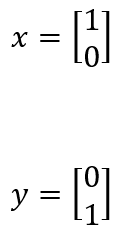Top number inside brackets is the x contribution to the basis-vector; bottom number is the y contribution to the basis-vector.

My velocity is equal to some number of these basis vectors: for instance, 50 of them in the y direction and 0 of them in the x direction. If we wanted to increase the number of them that represents my velocity, we would need to multiply the basis vectors by a number less than 1: for instance, I could just as easy say that I am moving at 100 half-kilometers per hour, but then I would be multiplying my basis vectors (the 1 km/h units) by half. This is known as contravariance (contra meaning opposite or against, and variance just meaning vary: multiplying the basis by smaller and smaller numbers causes the number of bases needed to represent my velocity to get bigger and bigger.

Well, since you are moving relative to me, your basis vectors, from your point of view, will look different to me. They will be rotated relative to me, and to calculate this, I will multiply my own basis vectors by something in order to rotate them around so that they align with your basis vectors. This is the linear transformation. If I wanted to, I could also change the magnitude of the basis vectors: for instance, if you were measuring your velocity in half-kilometers per hour while I was measuring mine in kilometers per hour. And so, I will need something that tells me exactly how much I’m changing the magnitude and the direction of my own basis vectors to transform them into your basis vectors. To do this, we use what is called a matrix. They can be arbitrarily big, but since we’re only talking about 2 dimensions, we need a 2-by-2 matrix.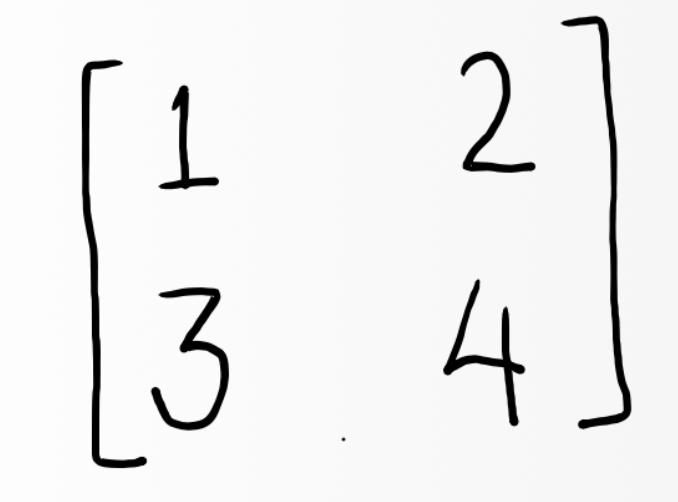Arbitrary numbers: doesn’t necessarily reflect the transformation I’m talking about in the article

When multiplying this by my basis vectors, the upper left tells me how much the magnitude of my x-basis vector needs to change to be the length of yours and the upper right tells me how much my x-basis vector needs to move in the y-direction to rotate it to be in the same direction as yours; the bottom left tells me how much my y-basis vector needs to move in the x-direction and the bottom right tells me how much the length of my y-basis vector needs to change.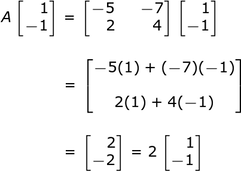Arbitrary numbers: doesn’t necessarily reflect the transformation I’m talking about in the article

This allows us to move from any particular frame of reference to any other particular frame of reference. But what are these matrices (plural of matrix)? These things have some properties, too. In the case of a 2×2 matrix, you can find what is the determinant by multiplying each diagonal and then adding the resulting products together: multiply the upper left with the bottom right and then subtract what you get for the product of the upper right and bottom left. This tells you, if you were to generate a parallelogram from the elements of the matrix, what the area of the parallelogram is (or volume for dimensions higher than 2). This is a measure of how much your vector is going to be scaled up when multiplied by the matrix. If the determinant is equal to one, then the transformed vector will have the same scale.The last thing we need to know about is what is called the Metric. Different Vector Spaces can have different Metrics. Metrics are used to define distance in your Vector Space. The Metric must have the following properties:

The distance from a point to itself is zero
The distance between two distinct points is positive
The distance from A to B is the same as the distance from B to A, and
The distance from A to B (directly) is less than or equal to the distance from A to B via any third point C.

What we use to determine the Metric (usually denoted g) is the sum of the multiplication of every basis vector from 1 frame of reference (point of view) with every basis vector from any other frame of reference (point of view). And so, in 2-dimensions, that would be:

g = X1*X2 + X2*Y1+ X1*Y2 + Y1*Y2

Which would be, for our 1 km/h basis vectors for the car example:

g = 1*1 + 1*0 + 0*1 + 1*1

And so we can represent g as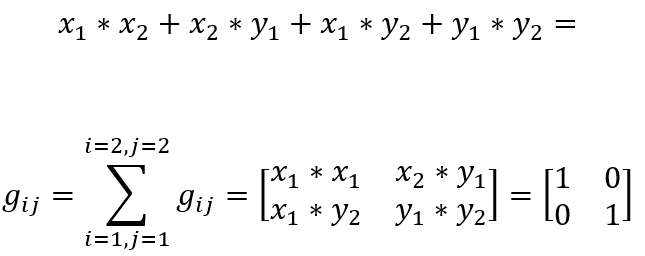Whereis the Euclidean Metric. The subscripts i and j on the g are indexes telling you what to sum and that big sigma sign is just telling you that you are summing on each of the indexes: that’s the subscripts in the X1*X2 + X2*Y1+ X1*Y2 + Y1*Y2.

You may also notice something else about this: where we have X1*X2 and Y1*Y2 is where it is not equal zero. And, if you recall your elementary algebra, x*x = x2 and y*y = y2 and if we add x2 + y2 then we get c2 which is

x2 + y2 = c2 = a2 + b2 = c2

And that’s the Pythagorean Theorem. This tells us that the Metric in Euclidean (flat) space is just the straight line – the Pythagorean Theorem tells us that using 2 sides of a right triangle gets us the length of the third side, which is equivalent to adding 2 component vectors to get the vector. Other sorts of space than just Euclidean Space have their own Metrics, for instance, 4-dimensional Minkowski Space (which is used for special relativity) has the Metric:Now we can talk about the further properties of Groups that will get us to Lie Groups

### Lie Groups

(Note before getting started for those who are more mathematically inclined: I am calling the elements of the Groups matrices, which I know gets into representation theory, but I think for the purposes of this simplified explanation, it’s good enough to just say that the elements are matrices).

To review, we said that a Group has to satisfy the following axioms:

A Group is closed
A Group has associativity
A Group has an identity element (like 0 for the integer numbers)
A Group is invertible (like a positive integer number having a negative integer number)

And we said that the Group can also be commutative. These properties can also apply to matrices: we can have a Group of matrices under the matrix multiplication operator. That means we can have a set of nxn matrices (e.g. 2×2 matrices) that

1. multiplying two nxn matrices together gives you another nxn matrix (e.g. if you multiply two 2×2 matrices you will get another 2×2 matrix)
2. if you multiply two nxn matrices A and B together, you get an nxn matrix C, which can then be multiplied by another nxn matrix D to get another nxn matrix F; also, you could multiplie B*C to get E and then A*E to get F
3. the identity element is the Metric: if you multiply any 2×2 matrix by the 2×2 Euclidean Metric, you will get the same matrix back
4. an nxn matrix A an be inverted into A-1 such that A*A-1 = I (the identity matrix)

This fourth axiom for matrices is slightly different for matrices of complex numbers and quaternions, in which case you need to use the transpose + complex conjugate, but I won’t get too into that. The inversion is calculated by swapping the positions of a and d, putting negatives in front of b and c, and dividing everything by the determinant (ad-bc):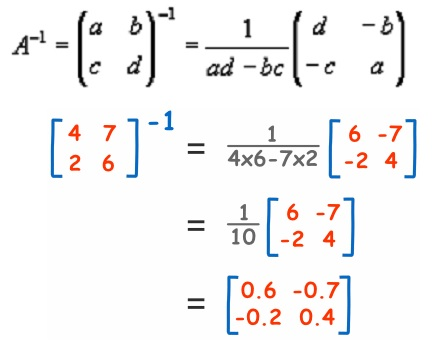However, with this idea of the transpose, if the transpose of a matrix and its inversion are equal, then it is orthogonal. The transpose is just flipping all the numbers over the diagonal that runs from top-left to bottom-right. And so, if you have the following matrix: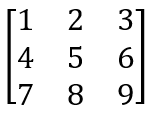Then the transpose would be:Where, as you can see, the diagonal from top-left to bottom-right stays where it is, but everything else is sort of mirror-reflected across that diagonal. And so, if the transpose is equal to the inversion, then the matrix is orthogonal. This means that if you use the matrix to transform orthogonal (perpendicular in 2 dimensions) vector spaces, you will maintain the orthogonality of the vector space. In 2D, that means if I transform from my frame of reference while driving the car to your frame of reference, the axes will remain perpendicular.

This gives us another kind of Group called the Orthogonal Group, denoted O(n) where n is the number of dimensions (so, for 2D it would be O(2) and for 3D it would be O(3) and so forth). This is our first Lie Group. This O(n) group is the set of all matrices that satisfy the 4 axioms listed above plus orthogonality (the inverse being equal to the transpose).

A property of this Orthogonal Group is that it preserves the Metric of any vector space that a Matrix element of the Group acts on. In other words, when I use a matrix from O(2) to transform from my frame of reference in my car to your frame of reference in your car, the Metric g remains the same.

Another property that a Group of matrices can have is that all of the matrices within the group can have a determinant of 1, which means that the matrices in the Group preserve the area (in 2D) or volume scale when making transformations. These are called SO(n) for Special Orthogonal group of n dimensions (as in SO(3) for Special Orthogonal group in 3 dimensions, for instance).

The SO(n) Groups have the property of being connected. What this means is that every matrix in the Group can be made from an infinitesimally small change in another matrix from the Group. For instance, if you have a vector on a 2D Cartesian coordinate plane that has a length of 1, there is a matrix that allows you to perform a transformation that rotates the arrow infinitesimally in the counterclockwise direction (we’ll call this infinitesimal rotation ϵ); there is also a matrix that would allow you to move it by 2ϵ, and another that would allow you to move it by 3ϵ, and so on. Therefore, we can think of the Group of matrices as being equivalent to a circle that goes all the way around the origin. This circle is continuous, since it is made up of all of the ϵ that get you from the starting point all the way back 360 degrees to the starting point.

(As an aside, the SU(n) Special Unitary group, which uses complex numbers, is more fundamental than the SO(n) group, since it is simply connected, and is the group used in physics. However, I didn’t want to complicate things by getting into complex numbers, but SO(n) and SU(n) are similar in a lot of ways, especially in the dimensions they are used for in physics).

This is one of the features of Lie groups is that they can form these shapes when we think of their elements as the topology they create. These topologies have mathematical properties, too, such as being a differentiable manifold – when the shape is plotted, you can perform calculus on it.

### Lie Algebra

The way that these shapes – the differentiable manifolds – are formed is not using the same Group operator as before. Before I told you that we an use regular matrix multiplication to combine two elements of the Group to get a third element of the Group. For Lie Groups, we use what’s called Lie Algebra. For Lie Algebra, instead of using normal matrix multiplication, we use what’s called the Lie bracket [ , ] which does the following operation:

[X,Y] = X*Y – Y*X

or, as a more concrete example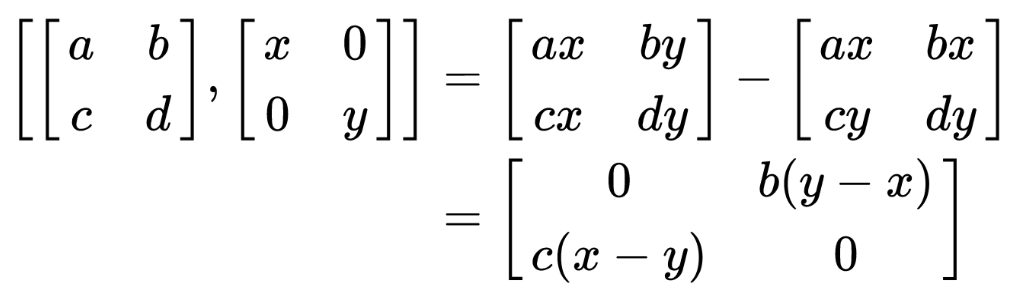We call these matrices X and Y are bases for the generators if they are able, through exponentiation with Euler’s Number, to generate all other elements in the Group. A generator is a set of basis matrices that can be combined, using the Group operator, to generate other matrices, which can then be combined with a basis matrix using the Group operator to generate other matrices, and so on until you have all of the matrices in the Group. This is analogous to the number 1 in the Group of integer numbers: you can combine 1 with 1 using the Group operator (addition) to get 2, then 2 with 1 to get 3, and then 3 with 1 to get 4 and so on, such that all integer numbers can be generated in this way.

What this looks like for our Lie Group is that, using the Group operator (the Lie Bracket) in exponentiated form can give us all other elements of the Group. This means:And the exponentiation of a matrix is: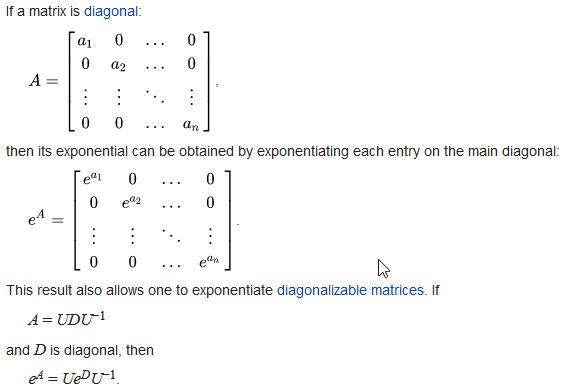And so the solution is:What this means is that, using the Lie Algebra, we can generate the entire Lie Group. The elements of the Lie Group are matrices which can be used to transform objects in vector space. The sorts of transformations that these matrices perform are those we observe in physics: 2-dimensional matrices in the SU(2) (similar to SO(3)) Lie Group describe the spin of particles; 3-dimensional matrices from the Poincaré Group (a type of 4-dimensional SO(n) group) describes motion in the Minkowski spacetime of special relativity.

What makes this interesting is that physical reality seems to care about the symmetries that create these Lie Groups. And, as Noether’s theorem tells us, all conserved values are really symmetries in disguise. And so, the symmetry of physical reality tells us that there are principles behind our physical laws: they aren’t haphazard.

That all being said, this is only a brief, oversimplified (and possibly inaccurate) overview of Lie Theory. If you see anything wrong with my interpretation, feel free to correct me.